Select Page

introduction

Discrete mathematics is a wide (and very active) area in mathematics which has become a central topic in modern research due to its deep interplay with theoretical computer science. The research of the combinatorics group exploits multiple connections of this area with many research disciplines including probability theory, number theory and group theory, among many others. Its interplay with logic and algorithimc plays also a strong line of research for our team.

More precisely, we aim to study several open problems inside the combinatorics framework, within the interplay between areas and in the interaction between different disciplines. In the first direction, we are interested in the study of random discrete structures, as random graphs and random sets, by the use of Erdös probabilistic method. The use of these probability techniques let us to study the typical behaviour of large random objects, as well as their limiting properties. Applications in real-world problems (as the comprehension of large networks) is also an important point addressed in this direction.

Concerning the interplay inside the research team, we are very interested on the role of pseudorandomness in computation. The hardness-vs-randomness trade-off of Nisan and Wigderson transformed the role of randomness in the design of algorithms. In this context, we investigate whether hardness for a model of symmetric computation could be enough to derandomize specific randomized constructions or algorithms. A celebrated example where the analysis of the randomness in a specific algorithm led to the breakthrough of the AKS algorithm, establishing that PRIMES is in P. another important line of research deals with the study of constrained classes of random graphs and logic limit laws: by using a combination of combinatorial, analytic, probabilistic and logical tools we are able to study the enumeration of planar graphs enriched with a global structure, and to determinine the set of limiting probabilities of graph properties in first-order logic for random graphs with given degree sequence; and analyzing the width of thresholds of random graph properties defined in suitable logical languages.

Finally, we are very much interested in the study of number theoretical problems (arising in abelian groups and in non-abelian groups) from a combinatorial perspective. This is the content of Arithmetic combinatorics: in the last years, there has been a dramatic growth of the area due to its connections with Ergodic Theory, Functional Analysis and Extremal Combinatorics. We aim to make progress on this area by combining a wide variety of modern combinatorial techniques (regularity methods, hypergraph containers, and arithmetic removal lemmas) to get a better understanding of maximal densities of sets avoiding certain patterns in groups (finite or infinite); and quasirandomness properties of the associated Cayley graphs, including the stationary behavior of random walks on these structures.

research lines

##### Logic & Algorithmics

Since its inception, mathematical logic has both contributed to and has been motivated
by the study of foundations of mathematics. Contemporary work in the foundations
of mathematics often focuses on establishing which parts of mathematics can be
formalized in particular formal systems. Algorithmics works in the description of the
set of steps that can be used to solve a specific computation and is becoming more
important in all mathematical fields

##### Combinatorics

Combinatorics is an area of mathematics concerned with the study of discrete
objects, for example, set systems, graphs, hypergraphs, integers, etc.. It is closely
related to many other areas of mathematics and has many applications including
logic, statistical physics and group theory, among others. Especially with theoretical
computer science, there is a rich interchange that has made this area a very active
field of modern mathematics.

members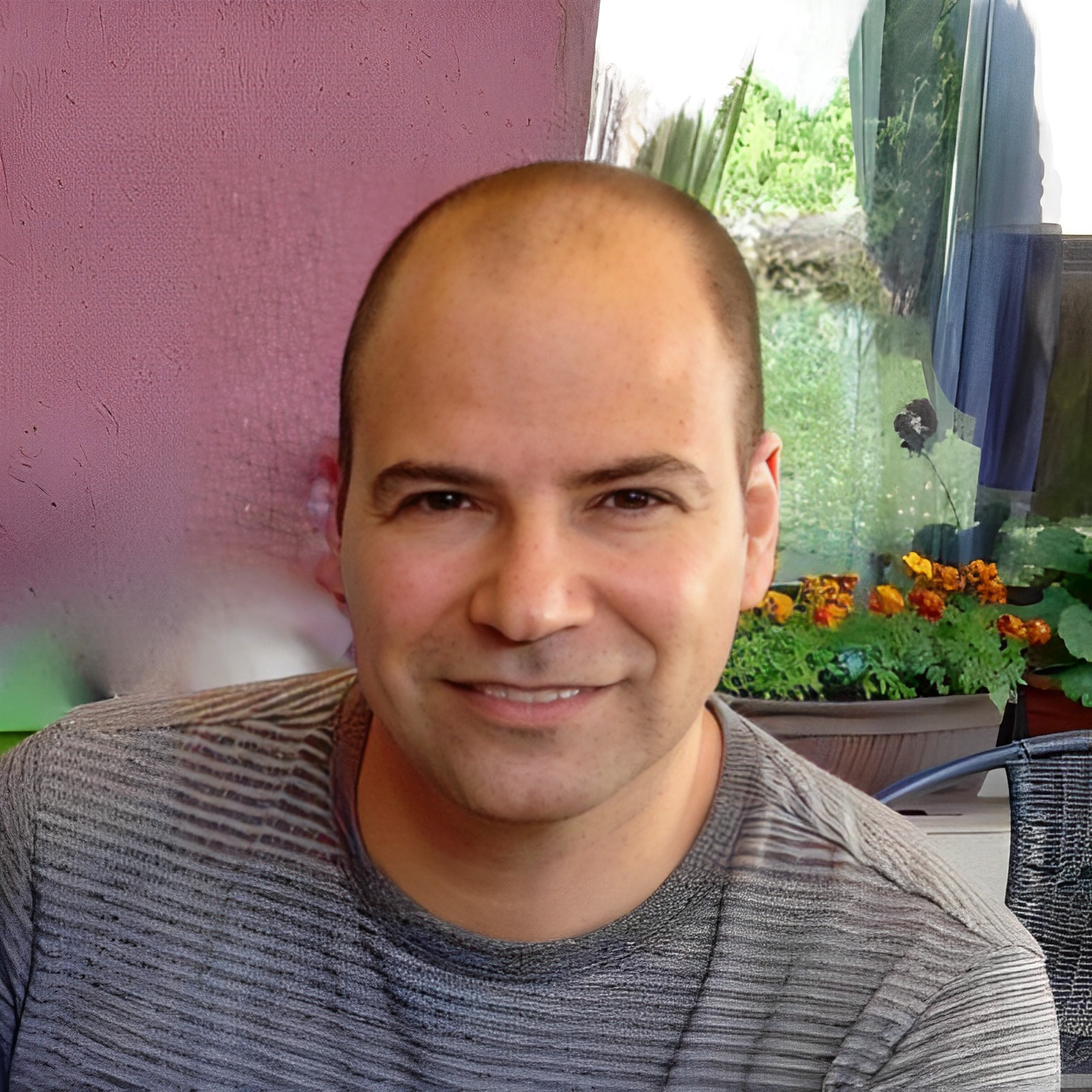WebsiteWebsite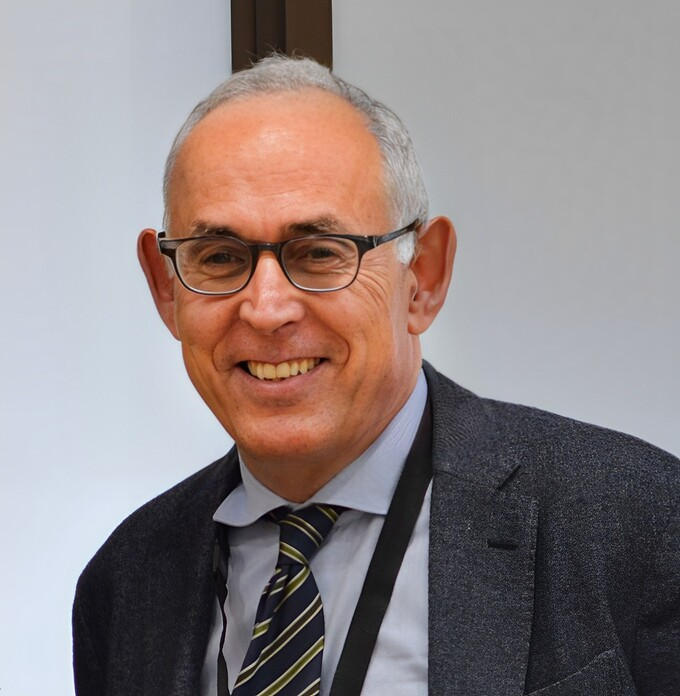Website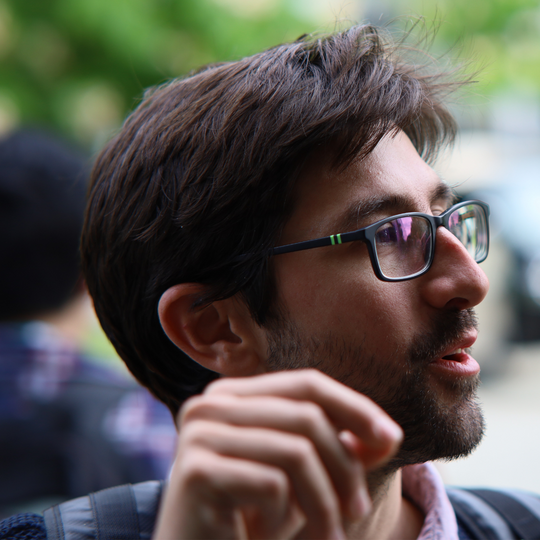Website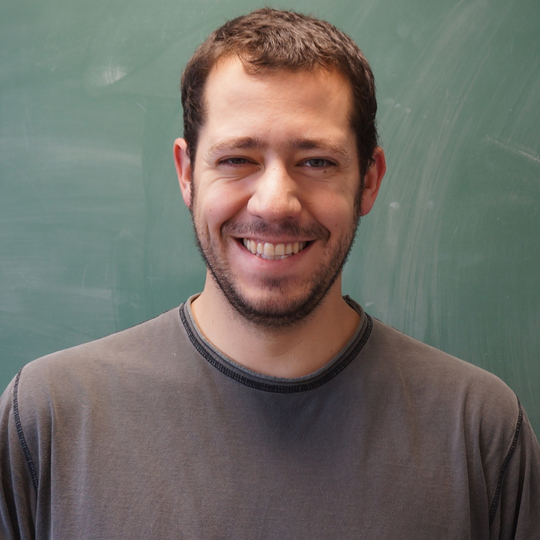Website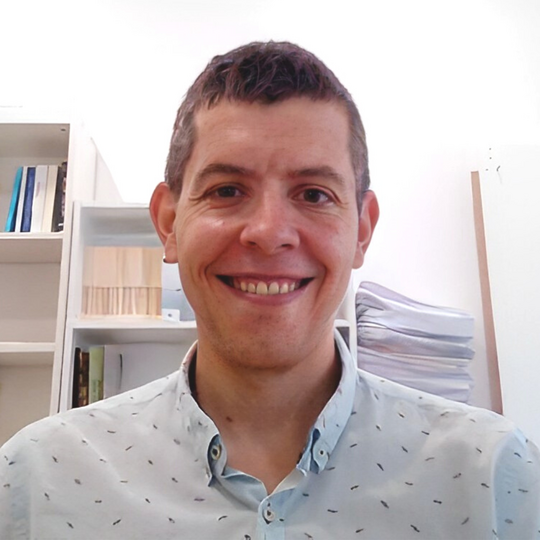WebsiteWebsite

postdoctoral researchers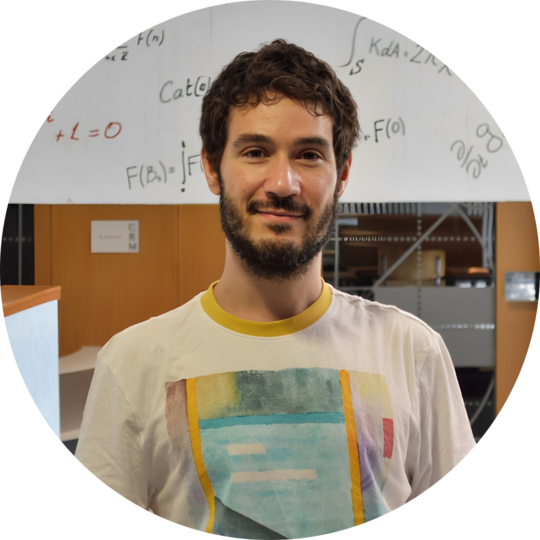#### Tássio Naia

Postdoctoral Fellow - CRM